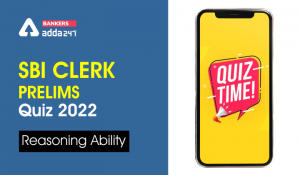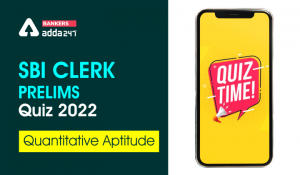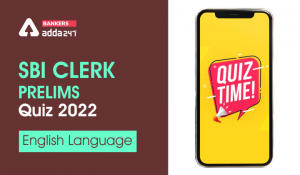Latest Banking jobs   »   Reasoning Ability Quiz For SBI Clerk...

# Reasoning Ability Quiz For SBI Clerk Prelims 2022- 26th April

Directions (1-5): Study the alphanumeric symbol series carefully and answer the questions given below.

4 1 \$ © 0 π 9 & £ 6 Ω @ 9 ≠ 2 7 ∞ 8 5 € # 6 µ 5 ¥ 4 ∑ 8 α 3 β 7 2 % 3

Q1. How many odd numbers are there between the third element from left end and fourth symbol from the right end?
(a) Two
(b) Three
(c) More than five
(d) Five
(e) None

Q2. Which of the following elements is exactly between the 10th element from left end and 12th element from right end?
(a) 2
(b) ∞
(c) 7
(d) 8
(e) None of these

Q3. If the last fifteen elements are reversed than which of the following is eleventh element to the left of the one which is fourth element from the right end?
(a) 5
(b) €
(c) 3
(d) %
(e) None of these

Q4. What is the sum of all the numbers between 11th element from left end and 8th element from right end?
(a) 50
(b) 51
(c) 48
(d) 46
(e) None of these

Q5. How many symbols are there which are immediately preceded by an even number and immediately followed by an odd number? (Consider Zero as an even number)
(a) Four
(b) Three
(c) More than four
(d) One
(e) None

Directions (6-10): Study the series of the letters carefully and answer the questions given below:

DOLF   EOMS   FDGH   TWIG   DIRB

Q6. If the first and the fourth letters of each word are interchanged, then how many meaningful words will be formed?
(a) Two
(b) One
(c) Three
(d) More than three
(e) None

Q7. If all the consonants are changed with their immediate preceding letter according to English alphabetical order in each word, then how many words will have two vowels?
(a) Two
(b) Three
(c) More than three
(d) One
(e) None

Q8. How many letters are there between the first letter of the word which is third from the left end and the second letter of the word which is third from the right end according to English alphabetical order?
(a) Two
(b) Four
(c) Six
(d) One
(e) More than six

Q9. If all the consonants are replaced with their immediate preceding letter and all the vowels are replaced with their immediate succeeding letter according to English alphabetical order then, how many vowels are there in the series?
(a) More than four
(b) Four
(c) One
(d) None
(e) Three

Q10. If all the words are arranged according to English alphabetical order from left to right, then which of the following is 4th word from the right end?
(a) DIRB
(b) EOMS
(c) DOLF
(d) TWIG
(e) FDGH

Directions (11-15): Study the number series carefully and answer the questions given below:

652   841   732   584   283

Q11. If one is subtracted from the third digit of the given numbers and one is added to the first digit of the given numbers, then which of the following will be the second-highest number?
(a) 283
(b) 584
(c) 732
(d) 652
(e) None of these

Q12. If the second and the third digits of each of the given numbers are interchanged, then how many odd numbers will be formed?
(a) Four
(b) Two
(c) One
(d) Three
(e) None of these

Q13. What will be the resultant, when the third digit of the third lowest number is multiplied with the second digit of the highest number?
(a) 15
(b) 6
(c) 8
(d) 12
(e) None of these

Q14. If all the numbers are arranged in ascending order from left to right, then which of the following will be the sum of all the three digits of the number which is second from the right?
(a) 21
(b) 10
(c) 15
(d) 12
(e) None of these

Q15. If all the digits in each of the given numbers are arranged in descending order within the number, then which of the following numbers will be the lowest?
(a) 283
(b) 841
(c) 652
(d) 584
(e) None of these

Solutions

S1. Ans. (d)
Sol. Clearly, five odd numbers are there between the third element from left end and fourth symbol from the right end.

S2. Ans. (b)
Sol.Clearly, ‘∞’ is exactly between the 10th element from left end and 12th element from right end.

S3. Ans. (c)
Sol.Clearly, 3 is eleventh element to the left of the one which is fourth element from the right end.

S4 Ans. (d)
Sol.Clearly, the sum of all the numbers between 11th element from left end and 8th element from right end is 46.

S5. Ans. (a)
Sol.0 π 9, 6 µ 5, 8 α 3 and 2 % 3
Clearly, four symbols are there which are immediately preceded by an even number and immediately followed by an odd number.

S6. Ans. (c)
Sol.After interchanging the first and fourth letters of each word
FOLD SOME HDGF GWIT BIRD
Clearly, three words are meaningful.

S7. Ans. (b)
Sol.After changing the consonants with their immediate preceding letter
COKE EOLR ECFG SVIF CIQA
Clearly, three words have two vowels.

S8. Ans.(d):
Sol.Clearly, there is only one letter between F and D according to English alphabetical order.

S9. Ans. (e)
Sol. After arrangement: CPKE FPLR ECFG SVJF CJQA
Clearly, there are only three vowels in the series.

S10. Ans. (c)
Sol.After arranging them according to English alphabetical order from right to left
DIRB DOLF EOMS FDGH TWIG
Clearly, DOLF is fourth word from the right end, if all the words are arranged according to English alphabetical order from right to left.

S11. Ans. (c)
Sol. After subtraction and addition – 751 940 831 683 382
Second highest number – 940 > 831 > 751 > 683 > 382
Therefore, option C is the correct answer.

S12. Ans. (b)
Sol. After interchange – 625 814 723 548 238
Odd numbers – 625 814 723 548 238
Therefore, option B is the correct answer.

S13. Ans. (c)
Sol. Given Series – 652 841 732 584 283
Resultant – 2 x 4 = 8
Therefore, option C is the correct answer.

S14. Ans. (d)
Sol. After arranging in ascending order – 283 584 652 732 841
Required sum = 7 + 3 + 2 = 12
Therefore, option D is the correct answer.

S15. Ans. (c)
Sol. After arranging in descending order – 652 841 732 854 832
Lowest number – 652
Therefore, option C is the correct answer.
umber = 753#### Congratulations!

Incorrect details? Fill the form again here

•Reasoning Ability Quiz For SBI Clerk Pre...
•Quantitative Aptitude Quiz For SBI Clerk...
•English Quizzes For SBI Clerk Prelims 20...
•Reasoning Ability Quiz For SBI Clerk Pre...
•English Quizzes For SBI Clerk Prelims 20...
•Reasoning Ability Quiz For SBI Clerk Pre...